Directions in Practical Lattice Cryptography Vadim Lyubashevsky IBM

• Slides: 58Directions in Practical Lattice Cryptography Vadim Lyubashevsky IBM Research – Zurich“Look back to where you have been, for a clue to where you are going. ” - ProverbThe Dark Ages ( 1978 – 1995) Similar to Five Dynasties and Ten Kingdoms period (after the Tang dynasty) A continuous circle of ad-hoc constructions followed by attacksKnapsack Problem a 1 a 2 … an t=Σaixi mod q Find xi t mod q xi in {0, 1}Vector Knapsack Problem a 1 a 2 … an t=Σaixi mod q Find xi t mod q xi in {0, 1}Vector Knapsack Problem a 1 a 2 … an t=Σaixi mod q t mod q xi “small” (<<q) Find xi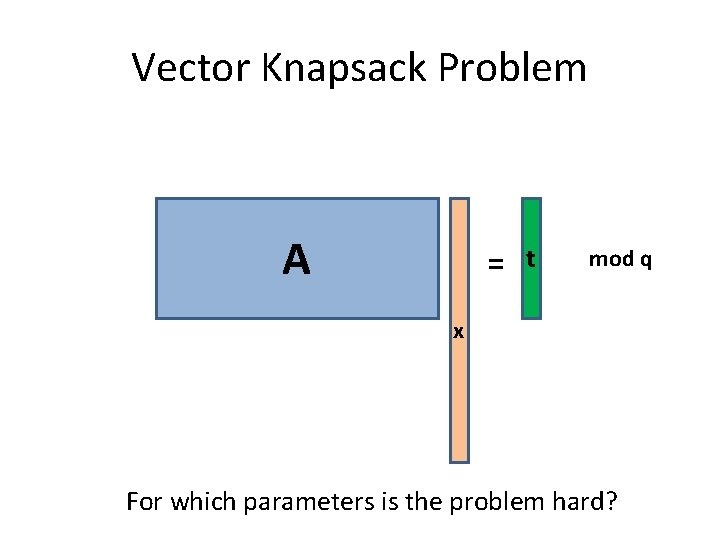Vector Knapsack Problem A = t mod q x For which parameters is the problem hard?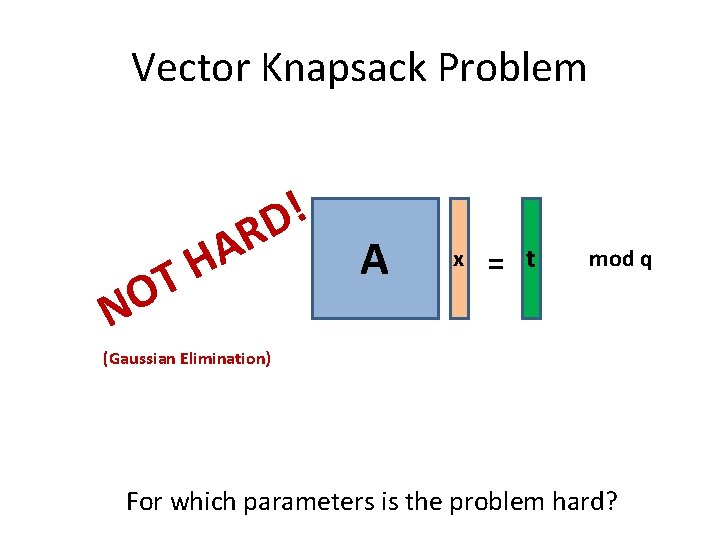Vector Knapsack Problem NO ! D R A H T A x = t mod q (Gaussian Elimination) For which parameters is the problem hard?Vector Knapsack Problem q is “exponentially” larger than xi A t mod q x R A H T O N ! D = (LLL and Lattice Reduction) For which parameters is the problem hard?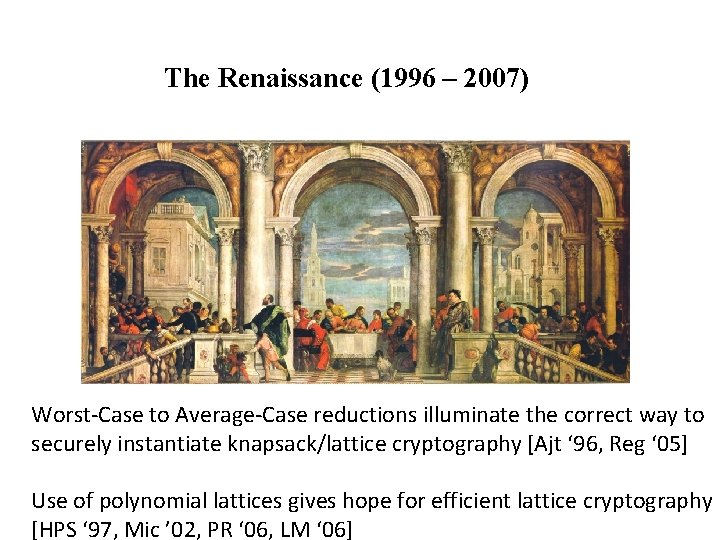The Renaissance (1996 – 2007) Worst-Case to Average-Case reductions illuminate the correct way to securely instantiate knapsack/lattice cryptography [Ajt ‘ 96, Reg ‘ 05] Use of polynomial lattices gives hope for efficient lattice cryptography [HPS ‘ 97, Mic ’ 02, PR ‘ 06, LM ‘ 06]Vector Knapsack Problem -1 B A B = x t -1 B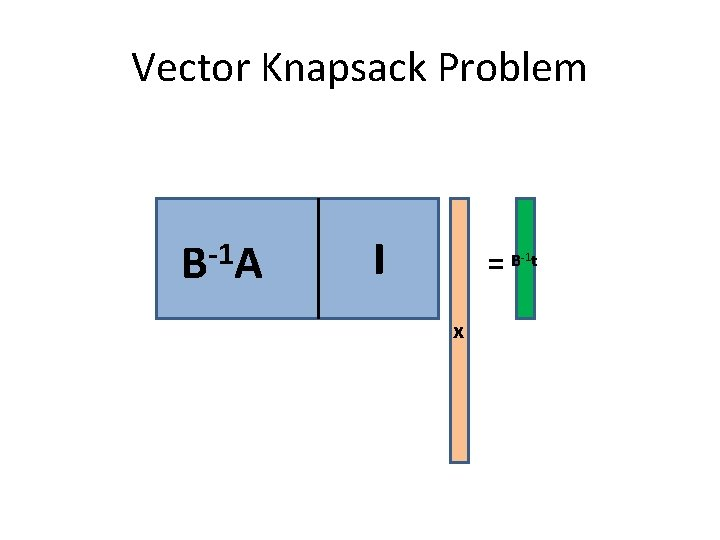Vector Knapsack Problem -1 B A I =B t -1 x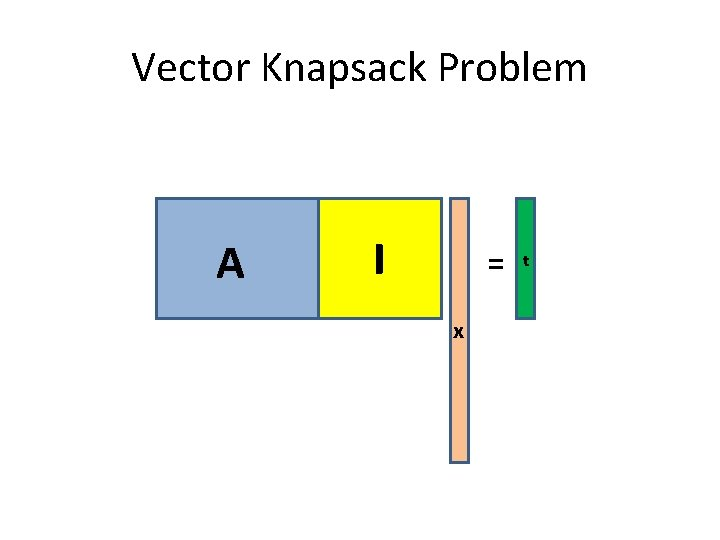Vector Knapsack Problem A I = x tLearning with Errors n A A A I t Regev [‘ 05]: I t Solving for x in this family of instances Finding short vectors in all lattices via a quantum algorithmn Learning with Errors I = t s + = tLearning with Errors n A s + e = t mod pGetting to the Beach in Hawaii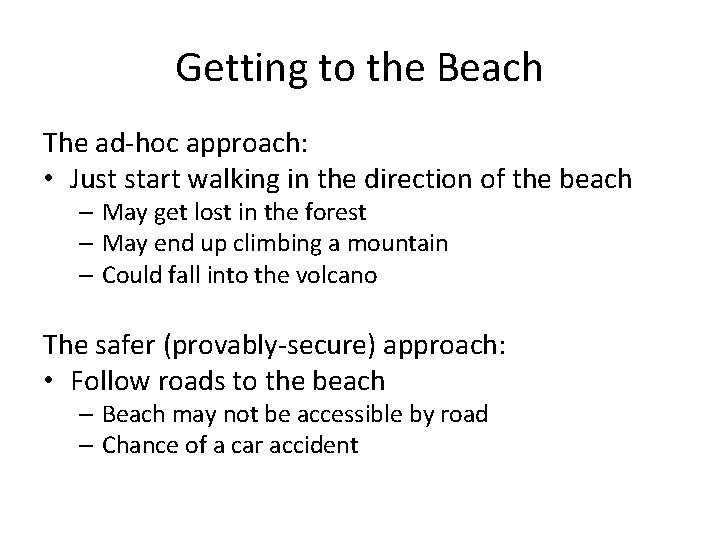Getting to the Beach The ad-hoc approach: • Just start walking in the direction of the beach – May get lost in the forest – May end up climbing a mountain – Could fall into the volcano The safer (provably-secure) approach: • Follow roads to the beach – Beach may not be accessible by road – Chance of a car accidentGetting to the BeachUsing Common Sense To get to the beach: 1. Use roads to get as close as possible to the beach 2. Get out of the car and try to find a safe way down To construct a secure public key scheme: 1. Get as close as possible using provable security 2. Try to make the scheme more efficient, without exposing it to attacks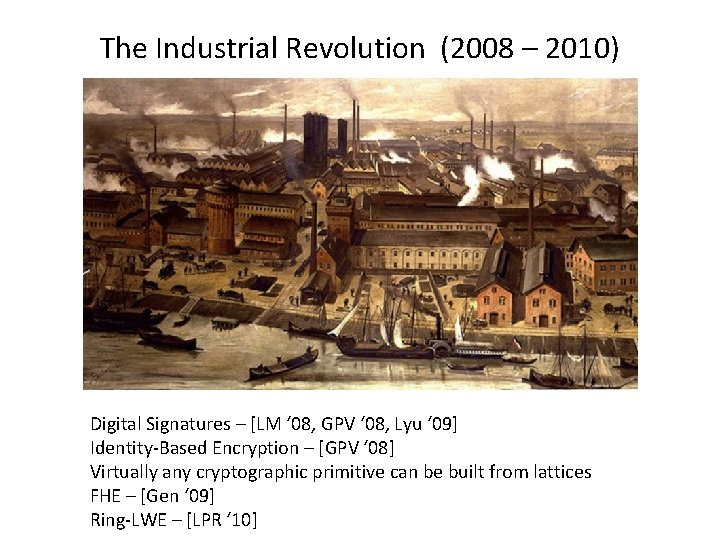The Industrial Revolution (2008 – 2010) Digital Signatures – [LM ‘ 08, GPV ‘ 08, Lyu ‘ 09] Identity-Based Encryption – [GPV ‘ 08] Virtually any cryptographic primitive can be built from lattices FHE – [Gen ‘ 09] Ring-LWE – [LPR ‘ 10]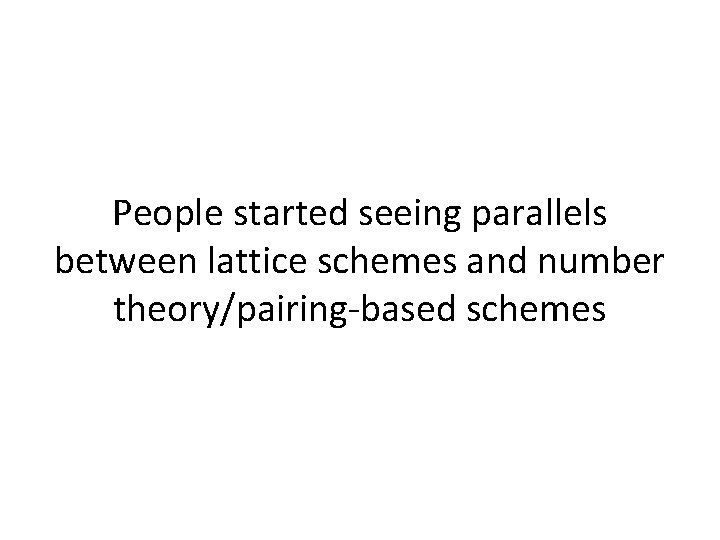People started seeing parallels between lattice schemes and number theory/pairing-based schemesDomains in Crypto Protocols “Discrete Log”: Hard problems in ring (Zp, +, *) for large p “Factoring” : Hard problems in ring (ZN, +, *) for N=pq Other domains?Polynomial Ring Zq[x]/(xn + 1) Elements are z(x)=zn-1 xn-1+ … +z 1 x+z 0 where zi are integers mod q Addition is the usual coordinate-wise addition Multiplication is the usual polynomial multiplication followed by reduction modulo xn+1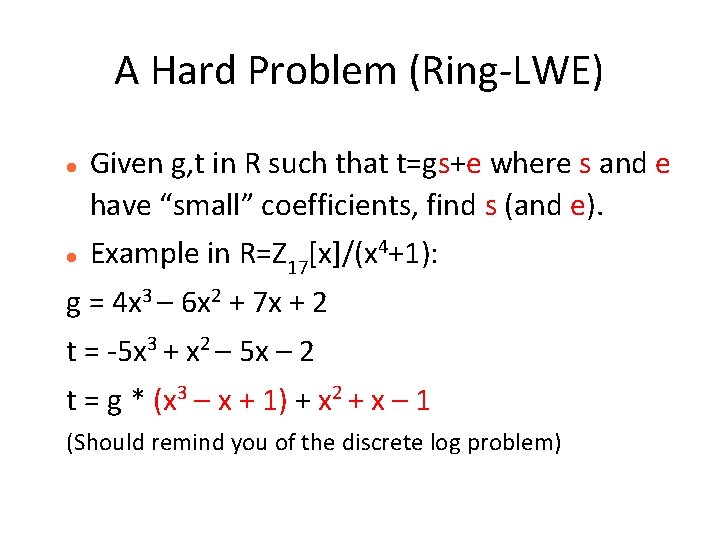A Hard Problem (Ring-LWE) Given g, t in R such that t=gs+e where s and e have “small” coefficients, find s (and e). Example in R=Z 17[x]/(x 4+1): g = 4 x 3 – 6 x 2 + 7 x + 2 t = -5 x 3 + x 2 – 5 x – 2 t = g * (x 3 – x + 1) + x 2 + x – 1 (Should remind you of the discrete log problem)The Decisional Version Given g, t in R, determine whether (1) there exist s and e with “small” coefficients such that t=gs+e or (2) g, t are uniformly random in R (Should remind you of the DDH problem)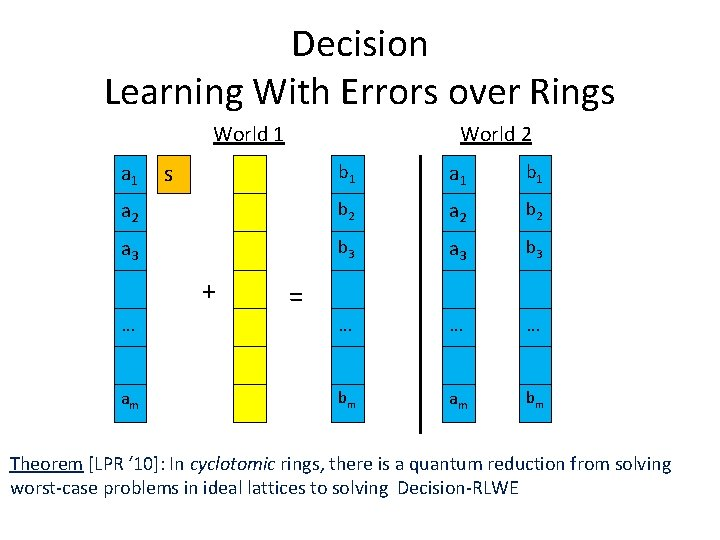Decision Learning With Errors over Rings World 1 a 1 World 2 s b 1 a 1 b 1 a 2 b 2 a 3 b 3 … … … bm am bm + … am = Theorem [LPR ‘ 10]: In cyclotomic rings, there is a quantum reduction from solving worst-case problems in ideal lattices to solving Decision-RLWE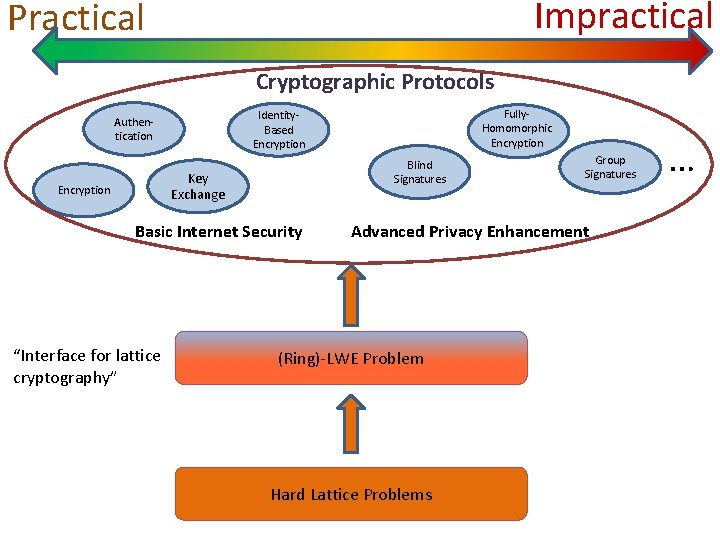Impractical Practical Cryptographic Protocols Blind Signatures Key Exchange Encryption Basic Internet Security “Interface for lattice cryptography” Fully. Homomorphic Encryption Identity. Based Encryption Authentication Group Signatures Advanced Privacy Enhancement (Ring)-LWE Problem Hard Lattice Problems …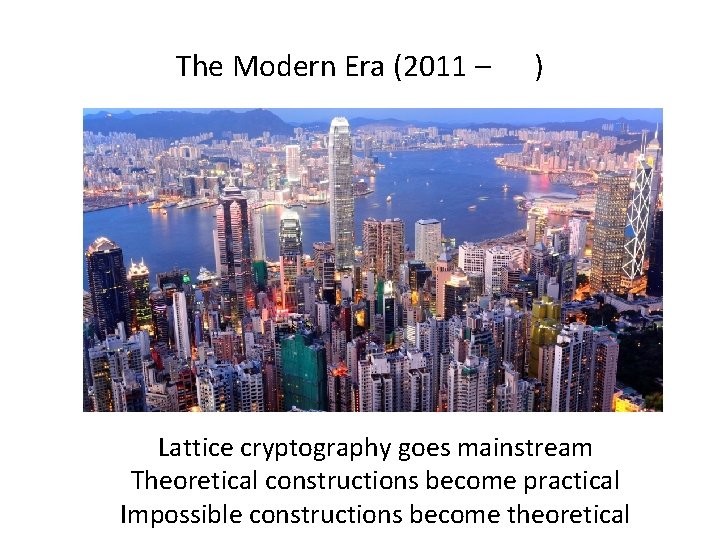The Modern Era (2011 – ) Lattice cryptography goes mainstream Theoretical constructions become practical Impossible constructions become theoreticalLWE Encryption n n S A E + = T Key mod p Generation r A Encryption T Encrypting b bits 0 u + e + = m Ciphertext Length: small Secret Key Length: can be very small S=H(s), E=H(e) Public Key Length: big no way to compress T v mod p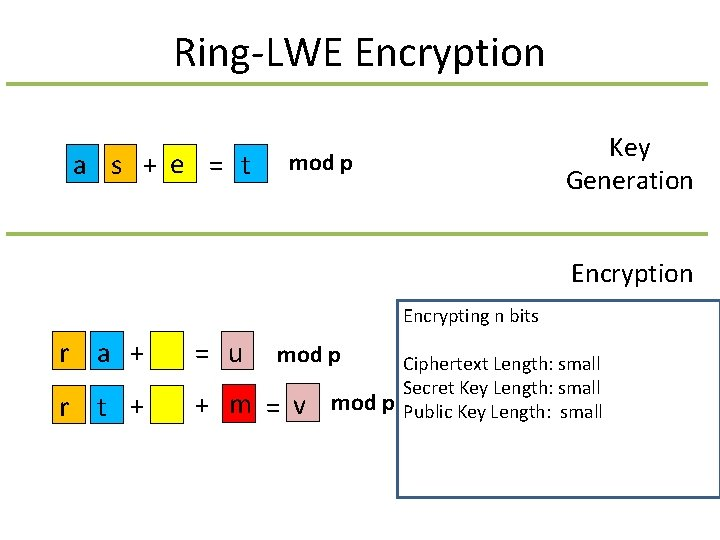Ring-LWE Encryption a s + e = t Key Generation mod p Encryption Encrypting n bits r a + = u r t + + m = v mod p Ciphertext Length: small Secret Key Length: small Public Key Length: small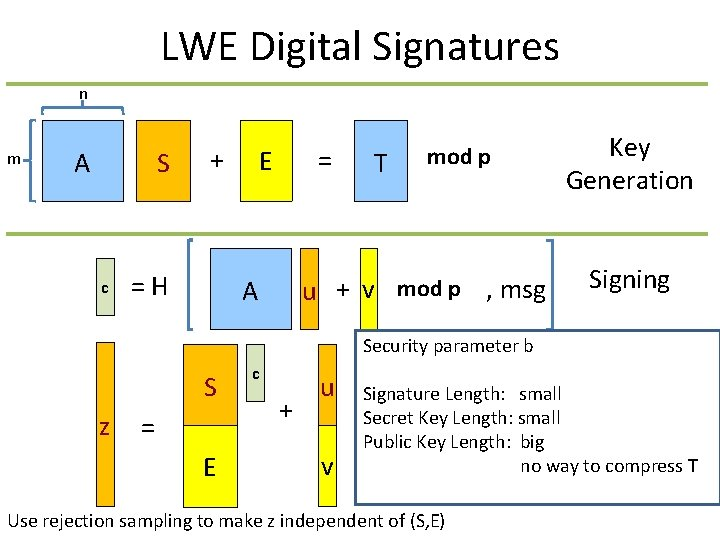LWE Digital Signatures n m S A c + = H = E T mod p u + v mod p , msg A Key Generation Signing Security parameter b S z = E c + u v Signature Length: small Secret Key Length: small Public Key Length: big no way to compress T Use rejection sampling to make z independent of (S, E)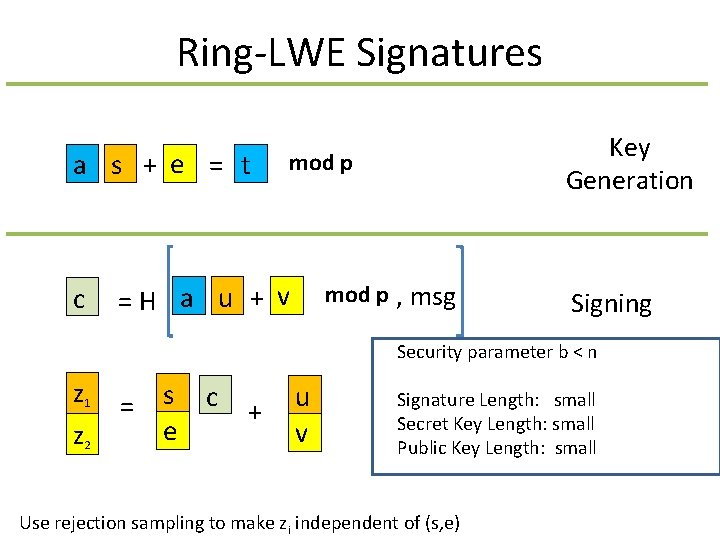Ring-LWE Signatures a s + e = t c Key Generation mod p = H a u + v mod p , msg Signing Security parameter b < n z 1 z 2 = s c e + u v Signature Length: small Secret Key Length: small Public Key Length: small Use rejection sampling to make zi independent of (s, e)Concrete Parameters 128 -bit quantum security Public Key Secret Key Output Size Encryption LWE: 200 – 400 KB LWE: < 1 KB LWE: 1 – 2 KB (of 256 bits) Ring-LWE: 1 – 2 KB Ring-LWE: < 1 KB Ring-LWE: 1 – 2 KB Signature LWE: 100 – 200 KB LWE: < 1 KB LWE: 1 – 2 KB Ring-LWE: 1 – 2 KB Ring-LWE: < 1 KB Ring-LWE: 1 – 2 KBGeneric Forward-Secure Authenticated Key Exchange from a 1 -Way KEM and a Signature vk vk (sk, pk) Key. Gen pk, Sign(pk) c, Sign(c) H(Decsk(c), View) = (c, m) Encpk(. ) H(m, View) Need pk, signatures, and ciphertext to be small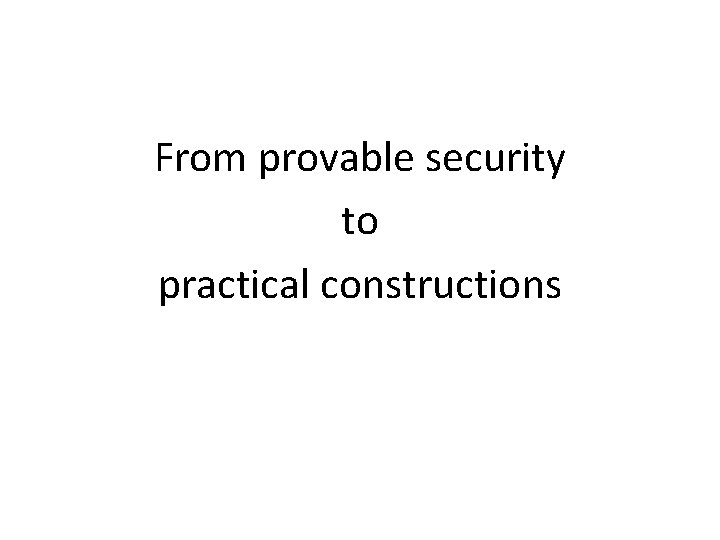From provable security to practical constructionsCase Study 1: (Ring)-LWE Encryption Secret Key a s + e = t r a + e 1 = u r t + e 2 + m = v Public Key For efficiency, want s, e, e 1, e 2 to be as small as possible. But [AG ‘ 11] says that if they are too small, then (Ring)-LWE is easy. But … the attack in [AG ’ 11] requires many linear equations – in the cryptosystem, we only have 2 n equations. So, is it safe to take very small (say 0/1) coefficients if q is not too large?Case Study 1: (Ring)-LWE Encryption Secret Key a s + e = t r a + e 1 = u r t + e 2 + m = v Public Key So, is it safe to take very small (say 0/1) coefficients if q is not too large? We thought so. And later, some evidence appeared • [MP ‘ 13] says that it is safe to use smaller LWE coefficients if there are few samples • [DM ‘ 13, MP ‘ 13] say that taking secret/errors from a non-Gaussian distribution is OK But these results apply to LWE, and not to Ring-LWE for technical reasons We still think it’s safe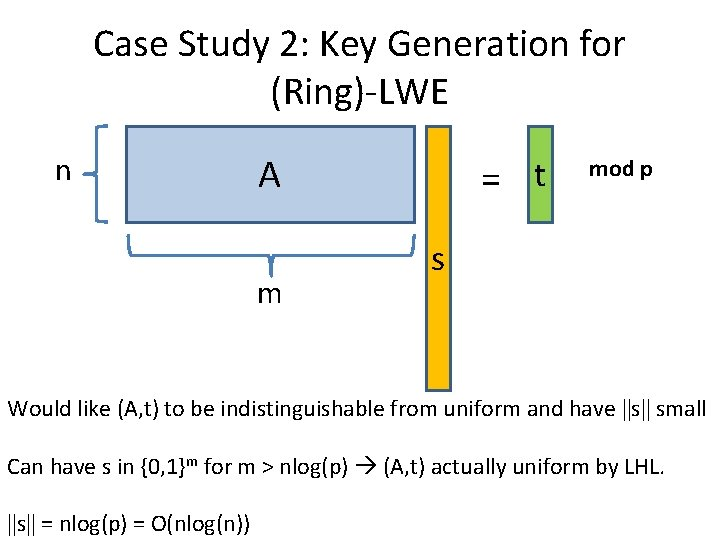Case Study 2: Key Generation for (Ring)-LWE n A m = t mod p s Would like (A, t) to be indistinguishable from uniform and have ||s|| small Can have s in {0, 1}m for m > nlog(p) (A, t) actually uniform by LHL. ||s|| = nlog(p) = O(nlog(n))Case Study 2: Key Generation for (Ring)-LWE n A I = t s 2 n mod p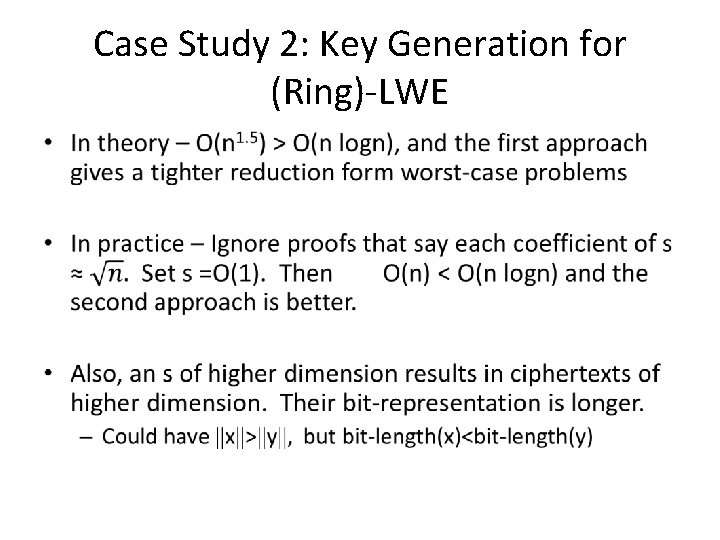Case Study 2: Key Generation for (Ring)-LWE •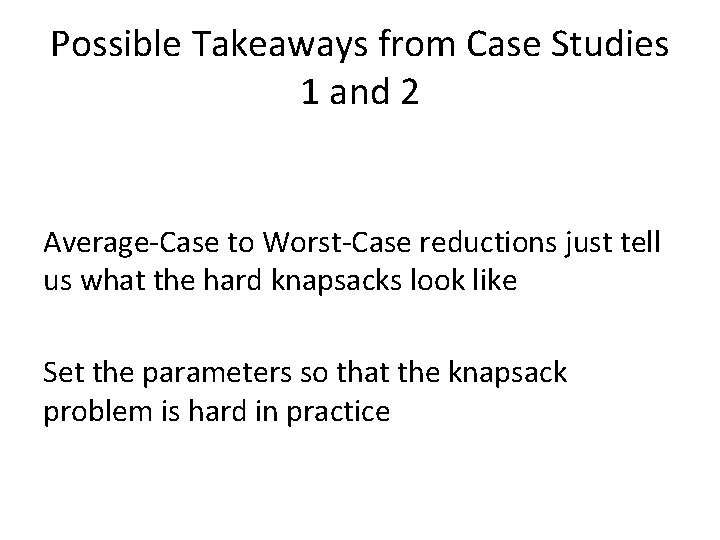Possible Takeaways from Case Studies 1 and 2 Average-Case to Worst-Case reductions just tell us what the hard knapsacks look like Set the parameters so that the knapsack problem is hard in practiceSetting Parameters n I A = x m t mod q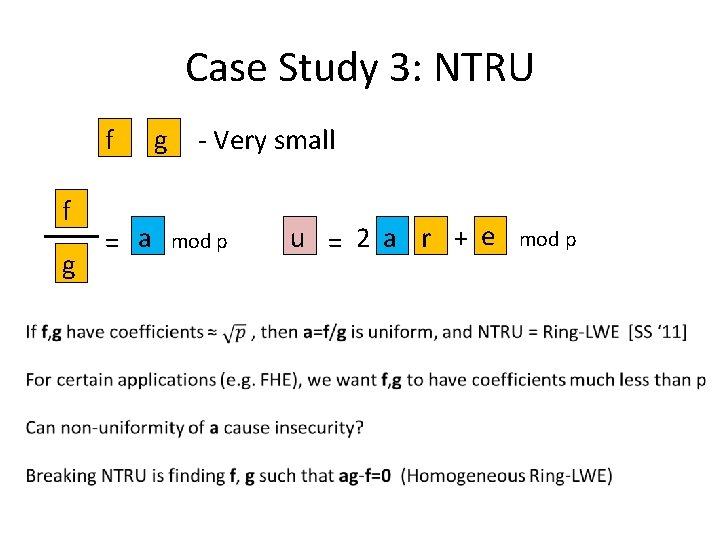Case Study 3: NTRU f f g = a g - Very small mod p u = 2 a r + e mod p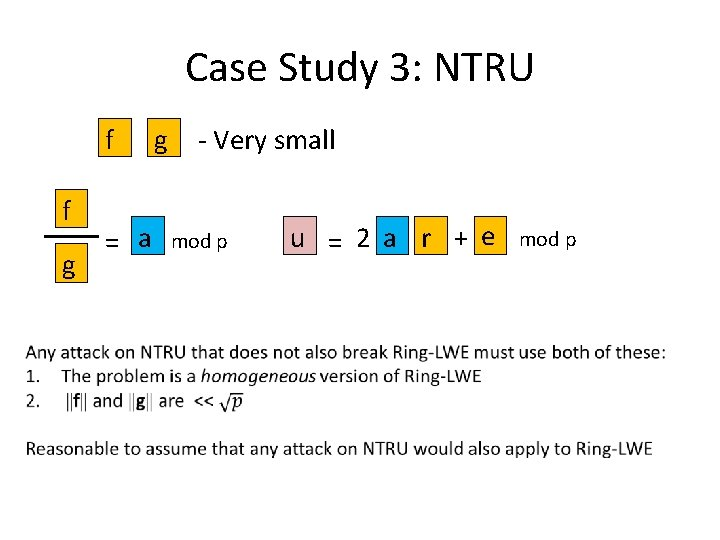Case Study 3: NTRU f f g = a g - Very small mod p u = 2 a r + e mod p“It isn’t what you don’t know that gets you into trouble. It’s what you know for sure that just isn’t so. ” - Mark TwainAttacking NTRU [ABD ’ 16, CJL ‘ 16] R=Z[x]/(xn+1) For any d | n, Subring of R: {a 0+a 1 xd+a 2 x 2 d+ … + an/d-1 xn-d : ai in Z, same operations as R} Such subrings of R are isomorphic to R’=Z[x]/(xn/d+1) The algebraic norm N: R R’ has the following properties: 1. For s, t in R, N(s)N(t)=N(st) 2. ||N(s)||<(||s||∙poly(n))dAttacking NTRU Idea for attacking NTRU. a=f/g N(a)N(g)-N(f)=0 mod p Lattice of dimension 2 n/d L={(g’, f’) : N(a)g’-f’=0 mod p} Find a short vector in this lattice – If ||(N(g), -N(f))|| is small, the solution will be a multiple of it. Then lift up to find (g, f).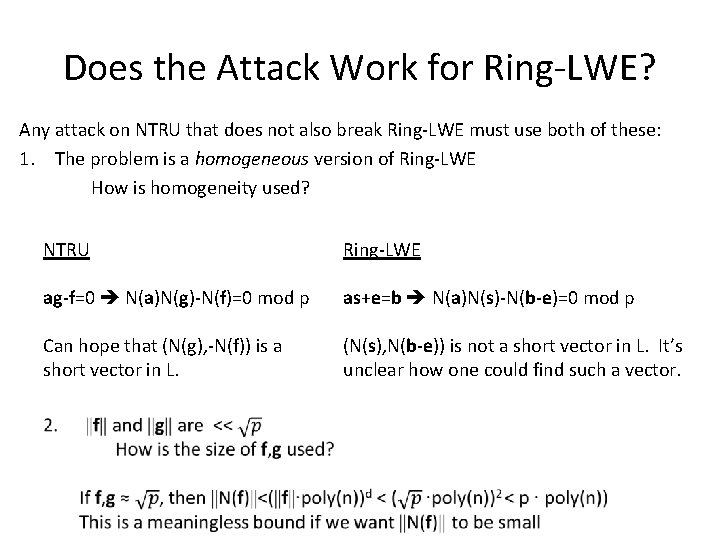Does the Attack Work for Ring-LWE? Any attack on NTRU that does not also break Ring-LWE must use both of these: 1. The problem is a homogeneous version of Ring-LWE How is homogeneity used? NTRU Ring-LWE ag-f=0 N(a)N(g)-N(f)=0 mod p as+e=b N(a)N(s)-N(b-e)=0 mod p Can hope that (N(g), -N(f)) is a short vector in L. (N(s), N(b-e)) is not a short vector in L. It’s unclear how one could find such a vector.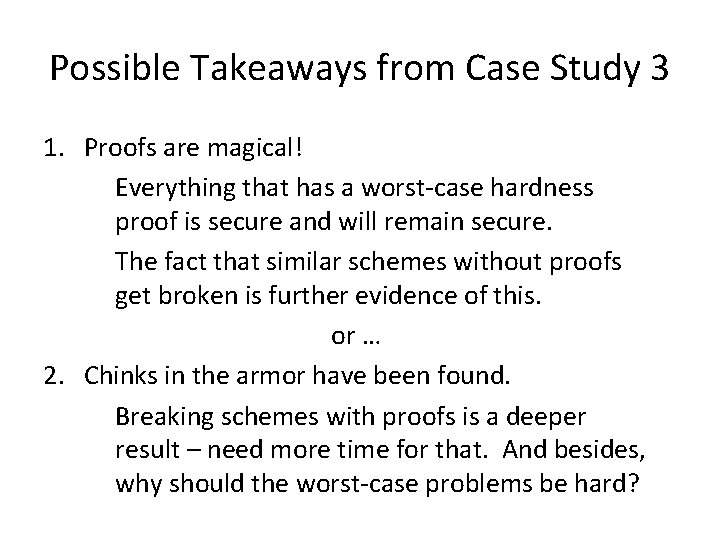Possible Takeaways from Case Study 3 1. Proofs are magical! Everything that has a worst-case hardness proof is secure and will remain secure. The fact that similar schemes without proofs get broken is further evidence of this. or … 2. Chinks in the armor have been found. Breaking schemes with proofs is a deeper result – need more time for that. And besides, why should the worst-case problems be hard?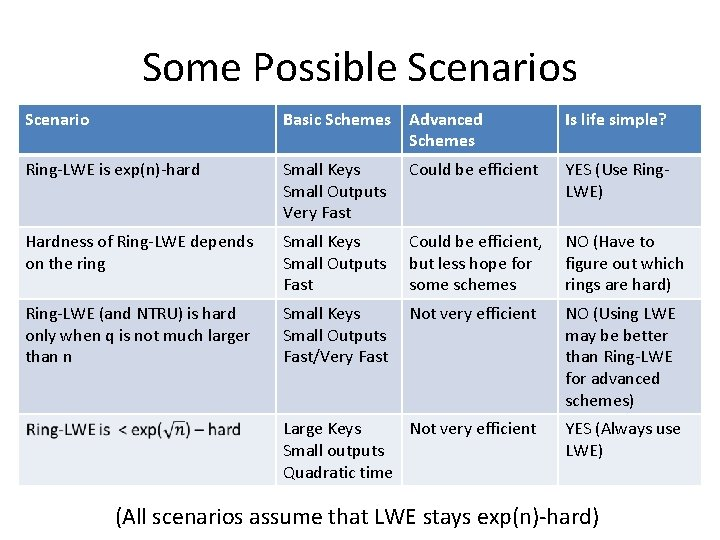Some Possible Scenarios Scenario Basic Schemes Advanced Schemes Is life simple? Ring-LWE is exp(n)-hard Small Keys Small Outputs Very Fast Could be efficient YES (Use Ring. LWE) Hardness of Ring-LWE depends on the ring Small Keys Small Outputs Fast Could be efficient, but less hope for some schemes NO (Have to figure out which rings are hard) Ring-LWE (and NTRU) is hard only when q is not much larger than n Small Keys Small Outputs Fast/Very Fast Not very efficient NO (Using LWE may be better than Ring-LWE for advanced schemes) Large Keys Not very efficient Small outputs Quadratic time YES (Always use LWE) (All scenarios assume that LWE stays exp(n)-hard)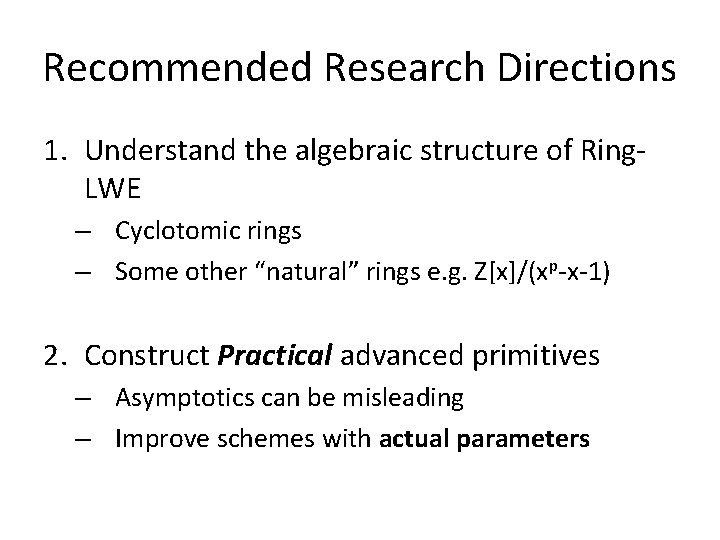Recommended Research Directions 1. Understand the algebraic structure of Ring. LWE – Cyclotomic rings – Some other “natural” rings e. g. Z[x]/(xp-x-1) 2. Construct Practical advanced primitives – Asymptotics can be misleading – Improve schemes with actual parameters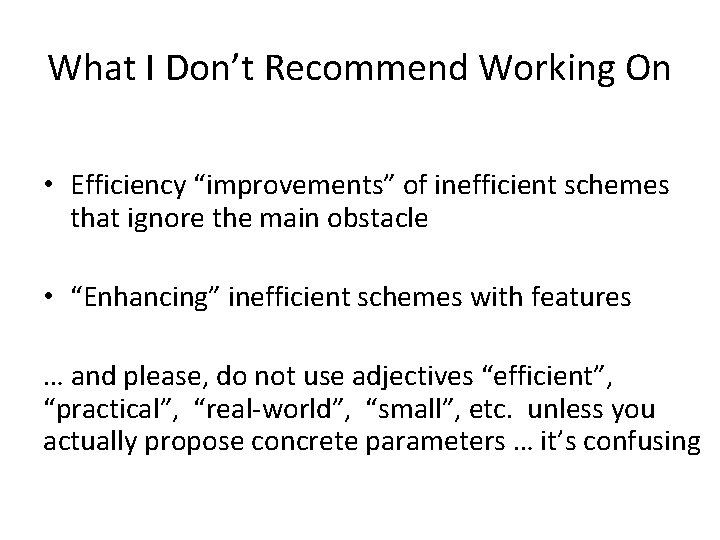What I Don’t Recommend Working On • Efficiency “improvements” of inefficient schemes that ignore the main obstacle • “Enhancing” inefficient schemes with features … and please, do not use adjectives “efficient”, “practical”, “real-world”, “small”, etc. unless you actually propose concrete parameters … it’s confusingIgnoring the Main Obstacle Getting closer to the edge of this cliff does not get you closer to getting to the waterAdding Features to Inefficient Schemes This is a solar-powered airplane Flight from Japan to Hawaii took 5 DaysA Submission to a Conference on “Post-Oil Transportation” Abstract In a seminal achievement, André Borschberg constructed a solar plane that flew from Japan to Hawaii in 5 days. In this work, we construct an equally efficient solar plane that additionally contains a touch-screen video-entertainment system. Because these devices are considered essential by today’s flying public, we believe that this is an important step towards the eventual mainstream adaptation of solar aircraft. This is silly, but happens in cryptography all the time.Conclusions • Lattice cryptography is very promising for basic quantumsafe schemes • Lattice cryptography is the only approach we know for advanced quantum-safe schemes • Definitely a topic that is worth researching, especially with NIST announcing a quantum-safe crypto contest • To build practical schemes, it is not enough to just work on “provably-secure” constructions – one needs to understand the underlying knapsack problemsThank You